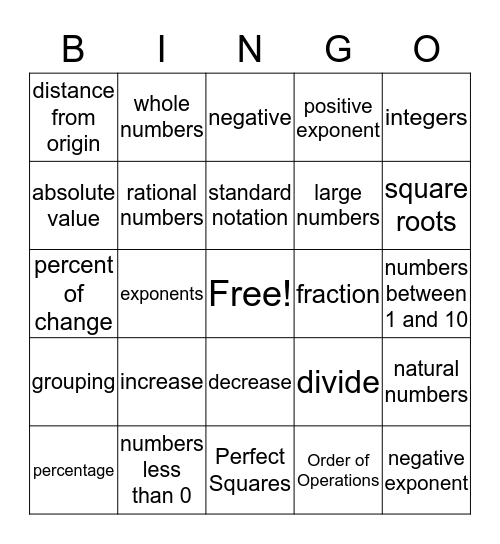# Units 1 & 2 of Transitional AlgebraThis bingo card has a free space and 32 words: Order of Operations, parenthesis, exponents, GEMDAS, grouping, rational numbers, integers, natural numbers, whole numbers, scientific notation, standard notation, negative, percent, decimal, fraction, order rational numbers, percentage, percent of change, decrease, increase, divide, cross-multiply, numbers between 1 and 10, numbers less than 0, negative exponent, positive exponent, large numbers, absolute value, distance from origin, Perfect Squares, formulas and square roots.

## Play Online